# Solving Systems of Linear Equations in Two Variables by Graphing

27 Oct 2017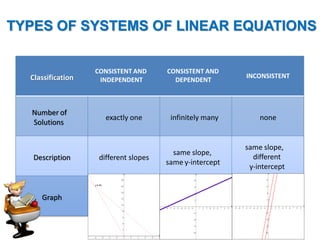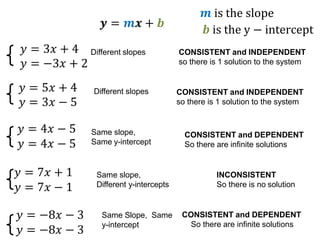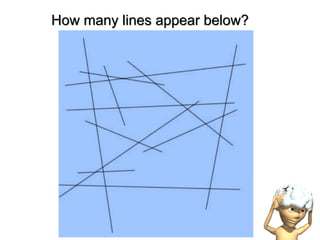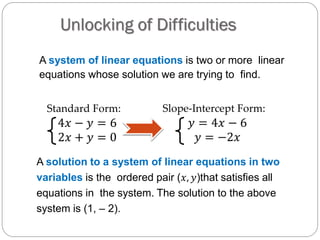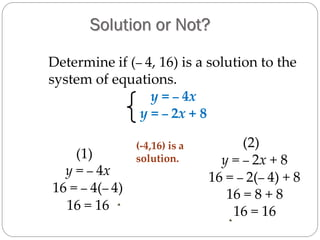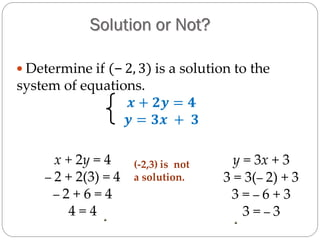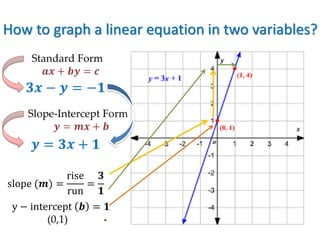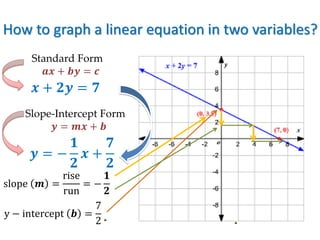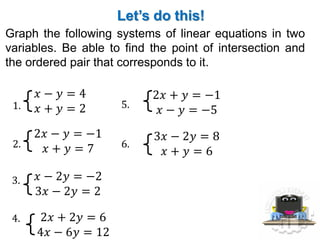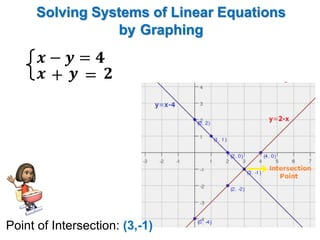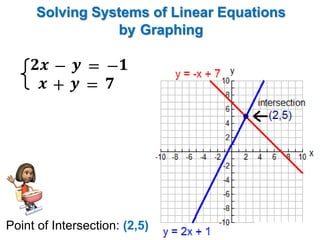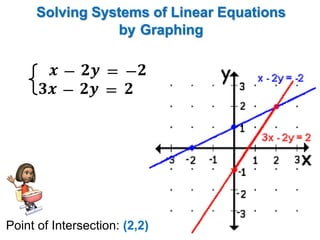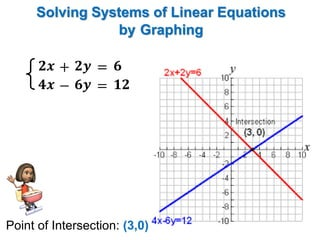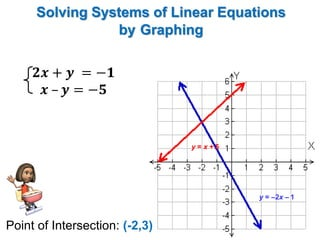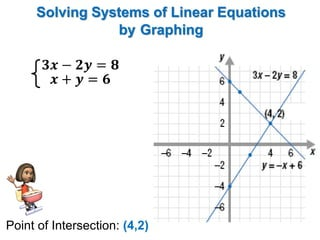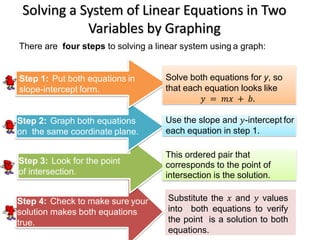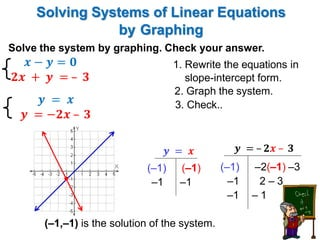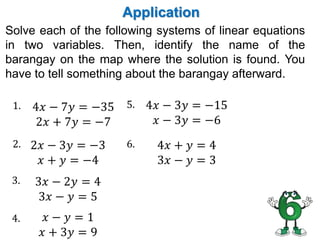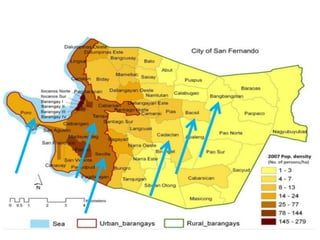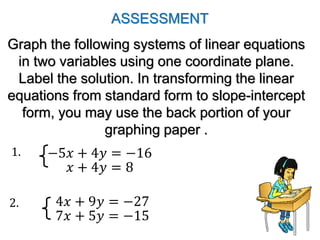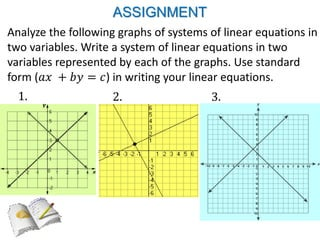1 sur 25

### Solving Systems of Linear Equations in Two Variables by Graphing

• 1. Solving Systems of Linear Equations in Two Variables by Graphing Presented by: JOEY F. VALDRIZ
• 2. LEARNING COMPETENCY: Solve a system of linear equations in two variables by graphing. Code: M8AL-Ii-j-1
• 3. RECALL What are the types of systems of linear equations in two variables?
• 4. TYPES OF SYSTEMS OF LINEAR EQUATIONS Classification CONSISTENT AND INDEPENDENT CONSISTENT AND DEPENDENT INCONSISTENT Number of Solutions exactly one infinitely many none Description different slopes same slope, same y-intercept same slope, different y-intercept Graph
• 5. 𝑦 = 3𝑥 + 4 𝑦 = −3𝑥 + 2 𝑦 = 4𝑥 − 5 𝑦 = 4𝑥 − 5 𝑦 = 7𝑥 + 1 𝑦 = 7𝑥 − 1 𝑦 = −8𝑥 − 3 𝑦 = −8𝑥 − 3 Different slopes CONSISTENT and INDEPENDENT so there is 1 solution to the system Different slopes Same slope, Same y-intercept Same slope, Different y-intercepts Same Slope, Same y-intercept CONSISTENT and INDEPENDENT so there is 1 solution to the system CONSISTENT and DEPENDENT So there are infinite solutions INCONSISTENT So there is no solution CONSISTENT and DEPENDENT So there are infinite solutions 𝑦 = 5𝑥 + 4 𝑦 = 3𝑥 − 5 𝒚 = 𝒎𝒙 + 𝒃 𝒎 is the slope 𝒃 is the y − intercept
• 6. How many lines appear below?
• 7. Unlocking of Difficulties A system of linear equations is two or more linear equations whose solution we are trying to find. A solution to a system of linear equations in two variables is the ordered pair (𝑥, 𝑦)that satisfies all equations in the system. The solution to the above system is (1, – 2). Standard Form: 4𝑥 − 𝑦 = 6 2𝑥 + 𝑦 = 0 Slope-Intercept Form: 𝑦 = 4𝑥 − 6 𝑦 = −2𝑥
• 8. (1) y = – 4x 16 = – 4(– 4) 16 = 16 (2) y = – 2x + 8 16 = – 2(– 4) + 8 16 = 8 + 8 16 = 16 (-4,16) is a solution. Determine if (– 4, 16) is a solution to the system of equations. y = – 4x y = – 2x + 8 Solution or Not?
• 9. Solution or Not?  Determine if (– 2, 3) is a solution to the system of equations. 𝒙 + 𝟐𝒚 = 𝟒 𝒚 = 𝟑𝒙 + 𝟑 x + 2y = 4 – 2 + 2(3) = 4 – 2 + 6 = 4 4 = 4 y = 3x + 3 3 = 3(– 2) + 3 3 = – 6 + 3 3 = – 3 (-2,3) is not a solution.
• 10. How to graph a linear equation in two variables? 𝟑𝒙 − 𝒚 = −𝟏 Standard Form 𝒂𝒙 + 𝒃𝒚 = 𝒄 Slope-Intercept Form 𝒚 = 𝒎𝒙 + 𝒃 𝒚 = 𝟑𝒙 + 𝟏 slope (𝒎) = rise run = 𝟑 𝟏 y − intercept 𝒃 = 𝟏 (0,1)
• 11. 𝒙 + 𝟐𝒚 = 𝟕 Standard Form 𝒂𝒙 + 𝒃𝒚 = 𝒄 Slope-Intercept Form 𝒚 = 𝒎𝒙 + 𝒃 𝒚 = − 𝟏 𝟐 𝒙 + 𝟕 𝟐 slope 𝒎 = rise run = − 𝟏 𝟐 y − intercept 𝒃 = 7 2 How to graph a linear equation in two variables?
• 12. Let’s do this! Graph the following systems of linear equations in two variables. Be able to find the point of intersection and the ordered pair that corresponds to it. 𝑥 − 𝑦 = 4 𝑥 + 𝑦 = 21. 2𝑥 − 𝑦 = −1 𝑥 + 𝑦 = 7 𝑥 − 2𝑦 = −2 3𝑥 − 2𝑦 = 2 2𝑥 + 2𝑦 = 6 4𝑥 − 6𝑦 = 12 2. 3. 4. 5. 6. 2𝑥 + 𝑦 = −1 𝑥 − 𝑦 = −5 3𝑥 − 2𝑦 = 8 𝑥 + 𝑦 = 6
• 13. 𝒙 − 𝒚 = 𝟒  𝒙 + 𝒚 = 𝟐 Solving Systems of Linear Equations by Graphing Point of Intersection: (3,-1)
• 14. 𝒙 + 𝒚 = 𝟕 𝟐𝒙 − 𝒚 = − 𝟏 Solving Systems of Linear Equations by Graphing Point of Intersection: (2,5)
• 15. 𝟑𝒙 − 𝟐𝒚 = 𝟐 𝒙 − 𝟐𝒚 = − 𝟐 Solving Systems of Linear Equations by Graphing Point of Intersection: (2,2)
• 16. 𝟒𝒙 − 𝟔𝒚 = 𝟏𝟐 𝟐𝒙 + 𝟐𝒚 = 𝟔 Solving Systems of Linear Equations by Graphing Point of Intersection: (3,0)
• 17. 𝟐𝒙 + 𝒚 = −𝟏 𝒙 – 𝒚 = −𝟓 y = x + 5 y = –2x – 1 Solving Systems of Linear Equations by Graphing Point of Intersection: (-2,3)
• 18. 𝟑𝒙 − 𝟐𝒚 = 𝟖 𝒙 + 𝒚 = 𝟔 Solving Systems of Linear Equations by Graphing Point of Intersection: (4,2)
• 19. Solving a System of Linear Equations in Two Variables by Graphing There are four steps to solving a linear system using a graph: Step 1: Put both equations in slope-intercept form. Step 2: Graph both equations on the same coordinate plane. Step 3: Look for the point of intersection. Step 4: Check to make sure your solution makes both equations true. Solve both equations for y, so that each equation looks like 𝑦 = 𝑚𝑥 + 𝑏. Use the slope and 𝑦-intercept for each equation in step 1. This ordered pair that corresponds to the point of intersection is the solution. Substitute the 𝑥 and 𝑦 values into both equations to verify the point is a solution to both equations.
• 20. Solve the system by graphing. Check your answer. 𝒚 = 𝒙 𝒚 = −𝟐𝒙 – 𝟑 1. Rewrite the equations in slope-intercept form. (–1,–1) is the solution of the system. 3. Check.. • 𝒚 = 𝒙 (–1) (–1) –1 –1 𝒚 = – 𝟐𝒙 – 𝟑 (–1) –2(–1) –3 –1 2 – 3 –1 – 1 2. Graph the system. 𝒙 − 𝒚 = 𝟎 𝟐𝒙 + 𝒚 = – 𝟑 Solving Systems of Linear Equations by Graphing
• 21. Application Solve each of the following systems of linear equations in two variables. Then, identify the name of the barangay on the map where the solution is found. You have to tell something about the barangay afterward. 4𝑥 − 7𝑦 = −35 2𝑥 + 7𝑦 = −7 1. 2𝑥 − 3𝑦 = −3 𝑥 + 𝑦 = −4 3𝑥 − 2𝑦 = 4 3𝑥 − 𝑦 = 5 𝑥 − 𝑦 = 1 𝑥 + 3𝑦 = 9 2. 3. 4. 5. 6. 4𝑥 − 3𝑦 = −15 𝑥 − 3𝑦 = −6 4𝑥 + 𝑦 = 4 3𝑥 − 𝑦 = 3
• 23. −5𝑥 + 4𝑦 = −16 𝑥 + 4𝑦 = 8 Graph the following systems of linear equations in two variables using one coordinate plane. Label the solution. In transforming the linear equations from standard form to slope-intercept form, you may use the back portion of your graphing paper . 4𝑥 + 9𝑦 = −27 7𝑥 + 5𝑦 = −15 ASSESSMENT 1. 2.
• 24. Analyze the following graphs of systems of linear equations in two variables. Write a system of linear equations in two variables represented by each of the graphs. Use standard form (𝑎𝑥 + 𝑏𝑦 = 𝑐) in writing your linear equations. ASSIGNMENT 1. 2. 3.
• 25. “Life is not linear; you have ups and downs. It’s how you deal with the troughs that defines you.” ~Michael Lee-Chin